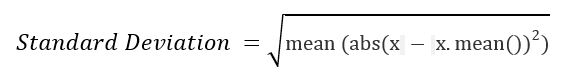# numpy.std() in Python

• Last Updated : 28 Nov, 2018

numpy.std(arr, axis = None) : Compute the standard deviation of the given data (array elements) along the specified axis(if any)..

Standard Deviation (SD) is measured as the spread of data distribution in the given data set.For example :

```x = 1 1 1 1 1
Standard Deviation = 0 .

y = 9, 2, 5, 4, 12, 7, 8, 11, 9, 3, 7, 4, 12, 5, 4, 10, 9, 6, 9, 4
Step 1 : Mean of distribution 4 = 7
Step 2 : Summation of (x - x.mean())**2 = 178
Step 3 : Finding Mean = 178 /20 = 8.9
This Result is Variance.
Step 4 : Standard Deviation = sqrt(Variance) = sqrt(8.9) = 2.983..
```

Parameters :
arr : [array_like]input array.
axis : [int or tuples of int]axis along which we want to calculate the standard deviation. Otherwise, it will consider arr to be flattened (works on all the axis). axis = 0 means SD along the column and axis = 1 means SD along the row.
out : [ndarray, optional]Different array in which we want to place the result. The array must have the same dimensions as expected output.
dtype : [data-type, optional]Type we desire while computing SD.

Results : Standard Deviation of the array (a scalar value if axis is none) or array with standard deviation values along specified axis.

Code #1:

 `# Python Program illustrating ``# numpy.std() method ``import` `numpy as np``   ` `# 1D array ``arr ``=` `[``20``, ``2``, ``7``, ``1``, ``34``]`` ` `print``(``"arr : "``, arr) ``print``(``"std of arr : "``, np.std(arr))`` ` `print` `(``"\nMore precision with float32"``)``print``(``"std of arr : "``, np.std(arr, dtype ``=` `np.float32))`` ` `print` `(``"\nMore accuracy with float64"``)``print``(``"std of arr : "``, np.std(arr, dtype ``=` `np.float64))`

Output :

```arr :  [20, 2, 7, 1, 34]
std of arr :  12.576167937809991

More precision with float32
std of arr :  12.576168

More accuracy with float64
std of arr :  12.576167937809991
```

Code #2:

 `# Python Program illustrating ``# numpy.std() method ``import` `numpy as np``   ` ` ` `# 2D array ``arr ``=` `[[``2``, ``2``, ``2``, ``2``, ``2``],  ``       ``[``15``, ``6``, ``27``, ``8``, ``2``], ``       ``[``23``, ``2``, ``54``, ``1``, ``2``, ], ``       ``[``11``, ``44``, ``34``, ``7``, ``2``]] `` ` `   ` `# std of the flattened array ``print``(``"\nstd of arr, axis = None : "``, np.std(arr)) ``   ` `# std along the axis = 0 ``print``(``"\nstd of arr, axis = 0 : "``, np.std(arr, axis ``=` `0``)) ``  ` `# std along the axis = 1 ``print``(``"\nstd of arr, axis = 1 : "``, np.std(arr, axis ``=` `1``))`

Output :

```std of arr, axis = None :  15.3668474320532

std of arr, axis = 0 :  [ 7.56224173 17.68473918 18.59267329  3.04138127  0.        ]

std of arr, axis = 1 :  [ 0.          8.7772433  20.53874388 16.40243884]
```

My Personal Notes arrow_drop_up